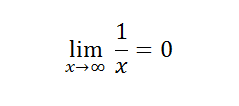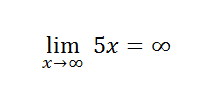# Limits Involving Infinity (Infinite Limits)

Limits involving infinity: contents :

## Limits Involving Infinity: Overview

Limits are a way to find answers for undefined problems in math such as 0/0 or ∞/∞. Since we can view limits as “what number will an equation approach?” and we can view infinity as a concept, we can match both of them in the concept of limits involving infinity.

The first thought that might come to mind is that 1/∞ is equal to zero. But, that isn’t mathematically correct. It’s not logically correct either, as this astute Quora respondent stated:

“If one divided by infinity equals zero, than that means [a] second divided by the infinity seconds that have already transpired equals zero, meaning you don’t actually exist.”

The real answer is that 1/∞ is very close to zero, but not quite. And that’s where limits come in: to give us reasonable answers to problems that are mathematically mind-boggling.

If we replace infinity with a variable x and give it large values, then this equation 1/x will be closer and closer to zero. We want to say that it will equal zero, but we can’t. This is where limits come to the rescue:

The limit of 1/x as x gets closer and closer to infinity equals zero.

In notation, that’s written as:## How to Solve Limits Involving Infinity: General steps

Since infinity can’t be used directly, we use limits. Let’s take a basic function:

y = 5x

The limit of this function when x approaches infinity is:As x gets nearer to infinity, the value 5x will also tend towards infinity. You’ll get the same result for:

• Any multiple of x,
• Any power of x,
• x divided by any number.

For example, the limit of all of these functions (as x gets larger and larger) equal infinity:

• x2,
• x3,
• 2x,
• 3x4,
• x/2,
• 40x3/600

## Signs

An important thing to look out when dealing with limits involving infinity is the sign before the variable x. If you’re dealing with a function that has –x or has –x raised to the highest power, then the answer will be “–infinity.” All positive functions will give positive limits.## Rational Functions

When dealing with rational functions, there are a few things to look out for. A simple rational function is f(x) = A(x)/B(x). Both A and B are polynomials . Let’s look at two examples:Here both A and B have the same degree of x. In this case, it’s 4. To get the limit, you only need to divide the coefficients in front of the variables with the highest exponent, to get 10/3.On the other hand, here we have the same situation. However, in front of one variable, we have a negative coefficient. The answer will also be the division of the two largest variables -9/4, but don’t forget the minus sign.

When working with limits involving infinity, if a function’s numerator has a higher power of the variable used, the answer will always be infinity.However, if the denominator (in our case B) is the one that has a higher exponent of the variable, the answer will always be zero.## Three Ways to Find Limits Involving Infinity

Infinite limits of functions are found by looking at the end behavior of functions. You can examine this behavior in three ways:

## A: Properties of Limits

Example problem: Find the limit at infinity for the function f(x) = 1/x.

There are a few handy “rules” we can use with limits involving infinity.

Check the Limit of Functions#properties”> Properties of Limits article to see if there’s an applicable property you can use for your function. Using a simple rule is often the fastest way to solve for a limit. If you don’t know how to use the rules, limits for polynomial functions explains the basic steps. For this example, there is a property for the function 1/xr. The property states that the limit is either positive or negative infinity:## B. Graph the function on a graphing calculator—like the TI89.Sometimes you’ll be able to see a clear trend just by looking at the graph. In this example, the graph above shows that as the function values increase, the y-values are clearly getting closer and closer to 0. Therefore, limx→ ∞1/x = 0.